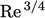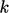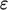## 6.15Summary of turbulence

• Turbulence is a spatially complex distribution of vorticity which advects itself in a chaotic manner, Sec. 6.2 .
• Vorticity is mostly generated at solid boundaries and evolves by advection, diﬀusion and stretching/compression, Sec. 6.3 .
• Boundary layers are thus the main source of vorticity for turbulence, Sec. 6.4 .
• Turbulence occurs when instabilities cause the vorticity to become chaotic, characterised by Reynolds Number, Sec. 6.1 .

### Scales of turbulence

• Length and time scales of turbulent eddies initially relate to the characteristic scales of the generated vortices, e.g. by shedding, Sec. 6.5 .
• The eddies become progressively smaller until they reach a size where the dissipation of their kinetic energy is signiﬁcant, Sec. 6.6 .
• The process of eddy breakup involves a cascade of kinetic energy from larger to smaller eddies in which eddy structures become increasingly isotropic, Sec. 6.7 .

### Simulating turbulence

• The Kolmogorov microscales represent the smallest scales of turbulence at which eddies die out by viscous dissipation.
• They are surprisingly small, e.g. a factor ofseparates the length scales of the largest and smallest eddies.
• Usually, it is prohibitively costly to capture the smallest scales in a CFD simulation, Sec. 6.8 .
• Large-eddy simulation captures the large eddies, while using modelling for the unresolved scales.
• Reynolds-averaged simulations provide faster solutions by solving equations for averaged ﬁeld variables, Sec. 6.9 .
• The averaged momentum equation includes the Reynolds stress which requires modelling.

### Turbulence modelling concepts

• Momentum exchange at the molecular scale is characterised by viscosity and kinetic theory provides good quantitative predictions of viscosity for gases, Sec. 6.10 .
• Comparing the random motion of eddies in a turbulent ﬂuid with particles at a molecular scale, Boussinesq introduced the concept of eddy viscosity, Sec. 6.11 .
• Eddy viscosity is not a ﬂuid property but is proportional to a characteristic speed and length scale of the turbulence; models are required which represent each of these scales.
• The speed scale is represented by turbulent kinetic energy, described by a transport equation Eq. (6.28 ), Sec. 6.13 .
• The-equation includes a term for its rate of dissipation; a transport equation for, e.g. Eq. (6.32 ), provides a model for that term — which also represents the length scale of turbulence, Sec. 6.14 .
• The concept of mixing length to characterise the length scale of turbulence provides additional modelling, particularly for the near-wall region, Sec. 6.12 .
Notes on CFD: General Principles - 6.15 Summary of turbulence